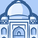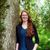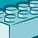# QlikView App Dev

Discussion Board for collaboration related to QlikView App Development.

cancel
Showing results for
Did you mean:Contributor II

## KPI and Ignore Filter Selection

Can I get some assistance with adjusting my code?

I want my KPI to ignore filters.
This is one situation where I miss Tableau. There you can simply check a box to let a filter work on an object or not.

=IF(SUM({<[Metric Name]={'XYZ'},[KPI Latest Month Figures]={'Yes'}>}Adjusted_Metric_Value)<0.001,'-',
ROUND(SUM({<[Metric Name]={'XYZ'},[KPI Latest Month Figures]={'Yes'}>}Adjusted_Metric_Value),0.1)&'%')

Thank you in advance.

Labels (3)

• ### KPI

1 Solution

Accepted SolutionsCreator

Hi,

When you want an expression not to consider the values ​​of a given filter, what you should do is include the name of the field not to be taken into account.

In the example attached, the expression is sum (sales), and the other expression ignores the Year filter.

sum (sales)                                      sum ({<year>} sales)

the set analysis {<year>}  indicate the year selections is ignored in the sum

5 RepliesCreator

Hi,

When you want an expression not to consider the values ​​of a given filter, what you should do is include the name of the field not to be taken into account.

In the example attached, the expression is sum (sales), and the other expression ignores the Year filter.

sum (sales)                                      sum ({<year>} sales)

the set analysis {<year>}  indicate the year selections is ignored in the sumMVP

In addition to the previous reply, if you want to ignore ALL filters, instead of listing out each one individually, you can simply add a 1 in the beginning of your set analysis:
=IF(SUM({1<[Metric Name]={'XYZ'},[KPI Latest Month Figures]={'Yes'}>}Adjusted_Metric_Value)<0.001,'-',
ROUND(SUM({1<[Metric Name]={'XYZ'},[KPI Latest Month Figures]={'Yes'}>}Adjusted_Metric_Value),0.1)&'%')Contributor II
Author

Yes, @gballester  "Year" is the particular filter that is interfering with my KPI.
Due to length of my formula, can you show me where to insert year?

After inserted {1} into my formula, realized I wanted to keep region filter working and have year deactivated.CreatorContributor II

Thanks! this is exactly what I was looking for